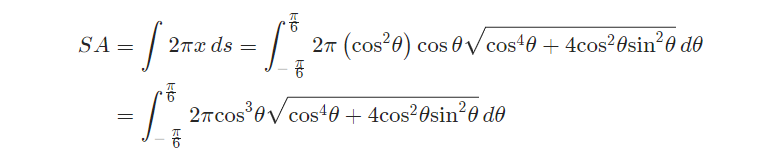### Still have math questions?

Algebra
Question

2. Set up, but do not evaluate, an integral that gives the surface area of the curve rotated about the given axis. You may assume that the curve traces out exactly once for the given range of $$\theta$$.

$$r = {\cos ^2}\theta$$$$\displaystyle - \frac{\pi }{6} \le \theta \le \frac{\pi }{6}$$ rotated about the $$y$$-axis.

The first thing we’ll need here is the following derivative.

$\frac{{dr}}{{d\theta }} = - 2\cos \theta \sin \theta$ Show Step 2

We’ll need the $$ds$$ for this problem.

$ds = \sqrt {{{\left[ {co{s^2}\theta } \right]}^2} + {{\left[ { - 2\cos \theta \sin \theta } \right]}^2}} \space d\theta = \sqrt {{{\cos }^4}\theta + 4{{\cos }^2}\theta {{\sin }^2}\theta } \space d\theta$ Show Step 3

The integral for the surface area is then,Remember to be careful with the formula for the surface area! The formula used is dependent upon the axis we are rotating about. Also, do not forget to substitute the polar conversion formula for $$x$$!

Solution
View full explanation on CameraMath App.